Previous: 7.2 The Open Von Up: 7.2 The Open Von Next: 7.2.2 Open System

## 7.2.1 Separation of Neumann Equation

For the Hamiltonian 6.2 the von Neumann equation for the density matrix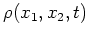reads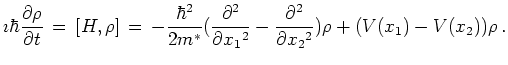(7.11)

To regain the Schrödinger equation from the von Neumann equation we make a separation ansatz for the density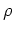: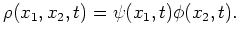(7.12)

We get (omitting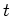in the function arguments)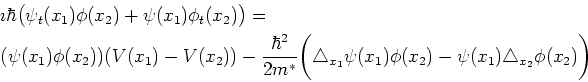(7.13)

Factoring out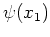and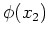gives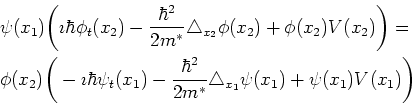(7.14)

Division by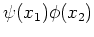separates the equation and we get (with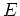as separation constant)(7.15)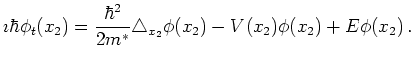(7.16)

If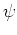is a solution to Equation 7.15 with separation constant, then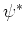is a solution to Equation 7.16 with complex conjugate separation constant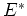and the density matrixis of the formcorresponding to a pure state.

We get an additional term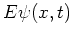in the separated equation, which is at first surprising since we are not in the stationary case. However, the von Neumann equation is invariant under a change of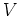to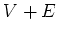, while the Schrödinger equation is not.

By separation of the transient von Neumann equation we get the Schrödinger equation with an additional term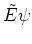. This shifts the value ofin the stationary Schrödinger equation. But varyingthe set of solutionsstays the same. So we can set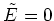without loss of generality.